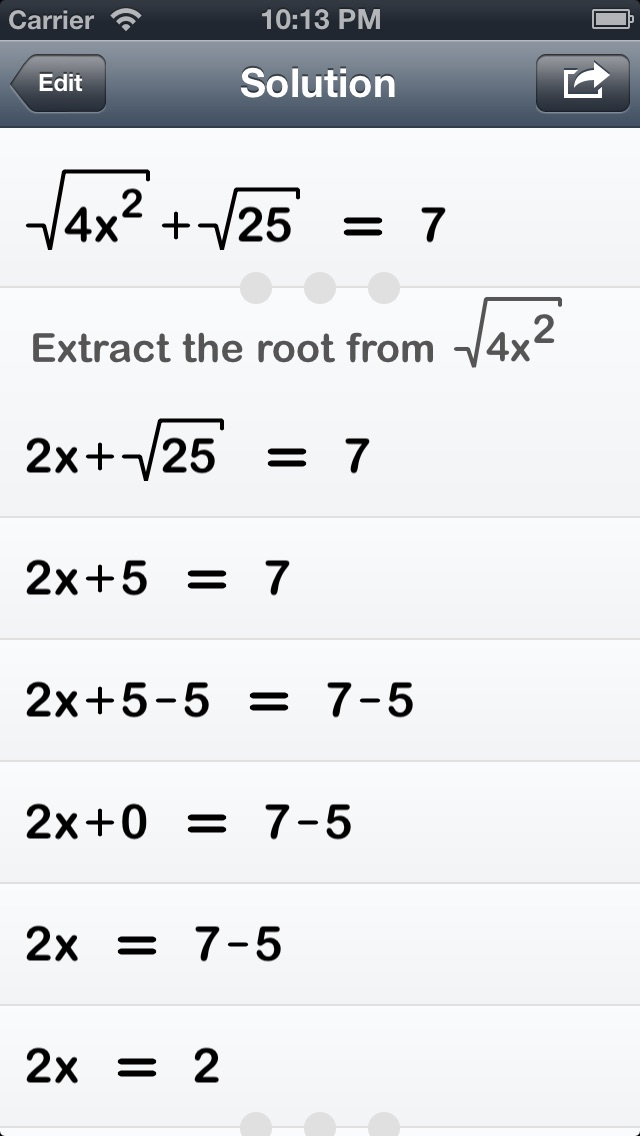# Polynom · more than just a calculatorPolynom is more than just a calculator. It solves your math exercises by providing simple step-by-step solutions. It is fast and simple to use, yet powerful by providing easy to follow explanations.

## Features

• Get simple solutions to math problems
• Detailed explanations for every single step
• Expand complicated steps to show every additional calculation step in all details
• Simplify terms, solve equations, find roots and much more## Behind the Scenes

The main goal of Polynom was to explain math problems in the most “human” way possible. In many cases, this requires picking one of many possible ways to solve a given problem in the most intuitive way.

To achieve this, a general goal-based problem solving engine was developed that imitates a human way of solving math problems. Goals (e.g. “Solve the given equation”) are achieved by repeatedly applying production rules to the current state of the problem which in turn might produce new intermediate goals (e.g. “Find the common factor”) until the original goal is reached.

As a side product, detailed descriptions are produced for each computation step that help to explain the solution.

Polynom is able to solve a wide range of primary and secondary school math problems, like solving linear and quadratic equations, finding roots, factoring fractions or dealing with simple exponentials. For this, almost 90 goals and 180 production rules are necessary.

Furthermore, the whole user interface—including the math input and output as well as the presentation of solutions, which offers the ability to expand and collapse sub-steps until and explanations for each step—is custom built.